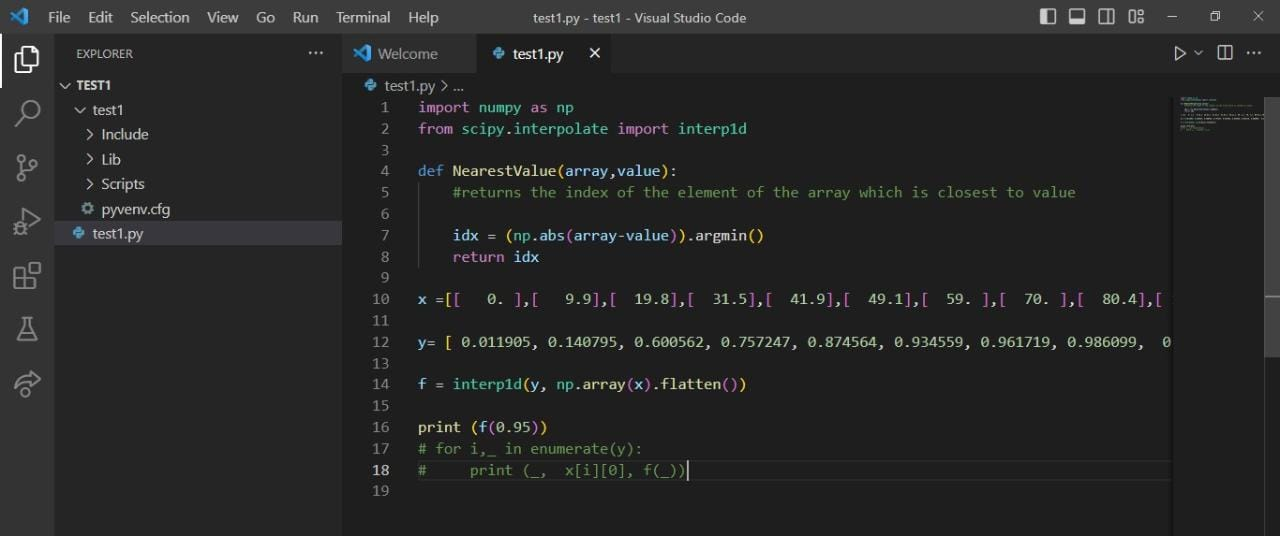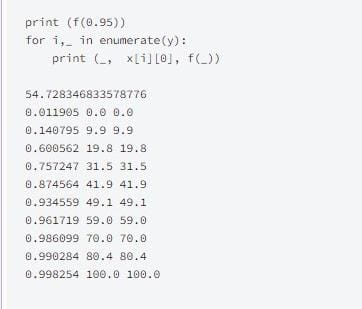# Interpolating a function using SciPy's interp1d functionby DejaswaroobaUpdated: Mar 24, 2023Solution Kit

A Python library called Scipy is intended for use in technical and scientific computing. It is based on the NumPy library and provides a range of mathematical tools and methods for uses in signal processing, interpolation, optimisation, and other fields. Together with Fourier transformations and image processing, Scipy also has modules for statistics and probability. Scipy is frequently used in conjunction with machine learning and data analytics in a variety of scientific fields, including physics, engineering, biology, and finance.

The Scipy subpackage interpolate offers a number of methods for interpolating data. Mathematical interpolation is a method for estimating the value of a function between two given values. It is helpful when we need to estimate values at places where there are no data or when our data points are not evenly spaced.

Scipy's interpolate subpackage offers a number of interpolation techniques, such as:

• interp1d: This function interpolates a one-dimensional data collection using linear and cubic spline interpolation.
• A two-dimensional data collection can be interpolated using bilinear and bicubic spline techniques using the interp2d function.
• griddata: This function interpolates a dispersed data collection onto an established grid.
• These functions, splev and splrep, allow for the assessment and creation of B-splines.

The scipy.interpolate module in Python's scipy library contains the inter1d function. It is employed for a function's one-dimensional interpolation.

The inter1d function's basic syntax is as follows:

f = interp1d(x, y, kind='linear'),

where x and y are arrays containing the data points and kind indicates the interpolation method to be used. The kind parameter can be set to "linear" to perform a linear interpolation, "nearest" to perform a nearest-neighbor interpolation, "cubic" to perform a cubic interpolation, or any other interpolation method.

The interpolated function can be evaluated at any location within the range of x after the inter1d function has been defined.Images depicting the functionality of the inter1d function.

### Code

In this program, linear interpolation is carried out using the interp1d function from the scipy.interpolate package.

• The NearestValue function uses the NumPy function argmin to determine the index of the element in the array that is closest to value.
• The code then generates an interp1d object f by using the interp1d function with the dependent variable y and the flattened independent variable x.
• The flatten() method is used to turn the list of lists x into a 1D array that the interp1d function requires.
• Finally, the code displays f(0.95), which is the interpolated value of the independent variable x that corresponds to the dependent variable value of 0.95. This signifies that the code is attempting to determine the value of x at which y equals 0.95 using linear interpolation.

• Copy and paste the above code from kandi in your prescribed code editor.
• Remove this part from the code.
•• The above just depicts the output received when we perform ennumeration, which is not necessary for interpolation.

### Dependent Libraries

scipyby scipy

Python11340Version:v1.11.0rc1SciPy library main repository

Support
Quality
Security
Reuse

scipyby scipy

Python11340Version:v1.11.0rc1License: Permissive (BSD-3-Clause)

SciPy library main repository
Support
Quality
Security
Reuse

If you do not have SciPy that is required to run this code, you can install it by clicking on the above link and copying the pip Install command from the SciPy page in kandi.

You can search for any dependent library on kandi like SciPy

### Environment tested

1. This code had been tested using python version 3.8.0
2. scipy version 1.10.1 has been used.

### Support

1. For any support on kandi solution kits, please use the chat
2. For further learning resources, visit the Open Weaver Community learning page.

See similar Kits and Libraries